﻿ The Credit Portfolio Management by the Econometric Models: A Theoretical AnalysisPublications are Open
Access in this journal
Article Versions
Export Article
• Normal Style
• MLA Style
• APA Style
• Chicago Style
Review Article
Open Access Peer-reviewed

### The Credit Portfolio Management by the Econometric Models: A Theoretical Analysis

International Journal of Global Energy Markets and Finance. 2018, 1(1), 1-3. DOI: 10.12691/ijgefm-1-1-1
Published online: April 17, 2018

### Abstract

This main idea of this paper is to examine theoretically the current model of credit portfolio management. We employ the credit portfolio view to examine the default probability measurement. The development of this type of model is based on a theoretical basis developed by several researchers. The evolution of their default frequencies and the size of the loan portfolio are expressed as functions of macroeconomic and microeconomic conditions as well as unobservable credit risk factors, which explained by other factors. We developed three sections to explain the different characteristics of this model. The purpose of this model is to assess the default probability of credit portfolio.

### 1. Introduction

The problem of evaluation of the failure probability of any borrower was the center of the bankers as soon as they began to lend some money. The quantitative modeling of the credit risk for a debtor is rather recent in fact. Besides, the modeling of the credit risk associated with instruments of a portfolio of credit such as, the loans, the pledges, the guarantees and the by-products (who constitute a recent concept).

A certain number of models were developed, including at the same time the applications of property developed for the internal custom by the financial institutions, and the applications intended for the sale or for the distribution 1.

The big financial institutions recognize his necessity, but there is a variety of approaches and rival methods. There are three types of models of credit portfolio in the course of use at present 2:

• The structural models: there are two models of management of credit portfolio who are supplied in the literature: Moody's KMV model (Portfolio Model) and CreditMetrics model by JPMorgan.

• The Macro-factors model (Econometric model): The Credit Portfolio View model introduces in 1998 by Mckinsey.

• The actuarial models CSFP (Credit Suisse First Boston): this model (CreditRisk+) is developed in 1997.

The main idea for this paper is to answer the question follows: How the default probability is defined by the credit portfolio models?

Then, the organization of this paper is as follows. In section 2, we define the econometric models and we define the main characteristics of the model Credit Portfolio View. We provide the forecast of the default rate in section 3. The section 4 is considered to present the conditional matrices of transition. We conclude in section 5.

### 2. The Econometric Models (Credit Portfolio View of Mackinsey)

Credit Portfolio View is a model with multiple factors which is used to feign the common conditional distribution of the default probability and migration for various groups of estimation and in different industries 2.

This model was developed by Wilson 3, 4 within McKinsey. The approach developed by this author bases itself on the hypothesis that the probability of defect and migration are connected to macroeconomic factors such as the level of the long-term interest rate, the growth rate of the GDP, the global unemployment rate, the exchange rates, the public spending, the savings.

Credit Portfolio View is based on the occasional observation which supposes that the default probability, as well as the probability of migration, is connected to economic cycles. When the economy is in situation of recession, then the cycles of credit are also lesser. If it is the opposite case (the economy is in situation of expansion) then the cycles of credit become stronger.

In other words the cycles of credit follow the tendency of economic cycles. Because the state of the economy is widely driven by macroeconomic factors, Credit Portfolio View proposes a methodology to connect these macroeconomic factors to the probability of default and migration.

Provided that the data are available, this methodology can be applied in every country, in the different sectors and in the diverse classes of borrowers of the obligors who react differently within the economic cycle.

The way that a model Credit Portfolio View works is as follows 5:

• Simulate the state of the economy.

• Adjust the rate of default to the state of the simulation of the economy.

• Attribute a probability of default for every debtor on the basis of the simulations of the state of the economy.

• The value of the individual transactions attributed to the debtors according to the probability of defect is determined on the basis of the simulations of the state of the economy.

• Calculate the loss of the portfolio by adding the results for all the transactions.

• Repeat all the stages quoted above certain number of times to map finally the distribution of the losses.

In the model Credit Portfolio View of McKinsey, the historic rates of default for the various industries are described according to the macroeconomic variables specified by the user of the model: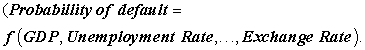In the approach McKinsey, the rates of defect are commanded by a sensibility in a sand of the factors of the systematic risk, or the specific factors to the company. The table below summarizes the main characteristics of the model of McKinsey 5:

### 3. The Forecast of the Default Rate

In the Credit Portfolio View model, the probabilities of default are modeled as being a Logit function. In this modeling the independent variable is a specific speculative index in every country and which depends on macroeconomic variables. The Logit function allows that the values of probability of default are included between 0 and 1 2, 6.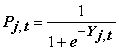and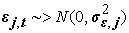.

Where,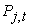indicate the conditional probability of default for period t for the debtors of the industry j andrepresent an indication stemming from a model in m factors.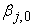,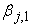, ...,are coefficients to be estimated by the method the Ordinary Last Squares (OLS).,, …,are values of economic variables in the date t of the industry or the country j.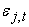represent a term of error which is normally distributed and independent of.

The model of McKinsey so land us land us note, as it is a model of macro-factors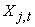who are represented by variable macroeconomic who follow a Autoregressive model of order 2 (AR2):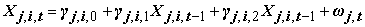and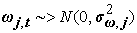Where:,and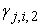are a coefficients to be estimated and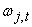is a term of error which is normally distributed and independent of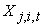.

In this frame, our objective is to resolve the system below: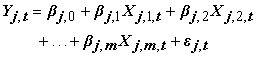Whereis the vector of the innovations such as: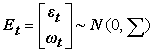and.

Where,andRepresent the matrices of correlation.

In case the parameters are estimated, then it is possible to feign the probability of default by basing itself on historical data. Credit Portfolio View uses tired matrices of transition of economic cycles.

### 4. The Conditional Matrices of Transition

By basing itself on the matrices of transition in the economic cycles which are proposed by the Credit Portfolio View, we can determine the situation of the economy 2. Noting in this respect that, the matrices of transition in the Credit Portfolio View are different to those of the matrices of migration in the CreditMetrics 6.

Credit Portfolio View proposes a tool based on the following ratio:Where,represent the probability of default feigned for date t and for the sector j andrepresent the historic default probability which is based on observed data.

If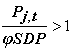then the economy is in period of recession and if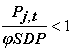then the economy is in period of expansion.

Credit Portfolio View suggests employing this ratio to adjust the probability of migration. So, the matrix of transition multi-period is given by the following formula:Where, M(.) can take two different values. So, M(.) = ML ifand M(.) = MH if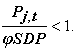Where, ML indicates the matrix of transition in the case of a period of recession and MH indicates the matrix of transition in the case of a period of expansion.

We can simulate a lot of time the matrix of transition to determine the probability of default for any estimation and for any period. The methodology of Monte Carlo Simulation can be used to determine the distribution of the default probability for any period.

The forces and the weaknesses relative to the Credit Portfolio View model are presented in Table 2.

### 5. Conclusion

In this paper, we present a theoretical approach’s concerning the model of management of credit portfolio by the Credit Portfolio View model.

The Credit Portfolio View model proposes a methodology which links macroeconomics factors to default and migration probabilities. The calibration of this model necessitates reliable default data for each country, and possibly for each industry sector within each country.

The probability of failure depends in these models of macroeconomic factors such as unemployment, the rate of increase GDP, the interest rate long-term.

### References

  Hickman, A. and Koyluoglu, H.U. (1998). A Generalized Framework Credit Risk Models. Working paper; an abridged version appeared as “Reconcilable Differences” Risk, vol. 11, no. 10, October 1998. In article  Crouhy, M., Galai, D. and Mark, R. (2000). A comparative analysis of current credit risk models. Journal of Banking & Finance, (24): 59-117. In article View Article  Wilson, T.C. (1997a). Portfolio credit risk (I), Risk, (10-9), 111-17. In article  Wilson, T.C., (1997b). Portfolio credit risk (II), Risk, (10-10), 56-61. In article  Smithson, C. (2003). Credit Portfolio Management. John Wiley & Sons. In article PubMed  Hamisultane, H. (2008). Modèles de gestion du risque de crédit. Investment System R&D, Document n°1. In articleThis work is licensed under a Creative Commons Attribution 4.0 International License. To view a copy of this license, visit http://creativecommons.org/licenses/by/4.0/